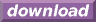#Calcute: freeware calculator

## Secant (sec)

The secant of an angle is defined using the right-triangle formed by this angle: it is the ratio of the triangle's hypotenuse to its adjacent side. Calcute implements it with the sec function.

```sec(1)
1.85081571768
```

In the above example, the given angle is in radians.

The triangle involved in the definition of the secant function is commonly shown in the cartesian plane with its adjacent side on the X axis and its angle point located at the origin (0,0). The other end of the triangle's hypotenuse marks a point on the periphery of a circle centered at the origin; the circle's radius is the length of the hypotenuse. If the angle is increased from 0 to 2 pi radians (0 to 360 degrees) then the end point of the hypotenuse traces a full circle. As the angle of the hypotenuse changes, so does its secant value as the shape of the corresponding triangle changes to match.

The secant is undefined at angle values that represent 1/4 and 3/4 of a full circle. At these angles, the triangle geometrically vanishes into a simple line segment on the Y axis. The value of the triangle's adjacent side is zero and division by zero is undefined. For example, sec is undefined at pi/2 radian, or equivalently at 90 degrees or at 100 gradiens.

The secant function is periodic. Except for those angles that match 1/4 or 3/4 of a circle, it is defined for all real numbers. As angle values increase (or decrease) outside the range of [0, 2 pi] radians that represent one full rotation, the value of sec for these angles repeats itself periodically with each new rotation.